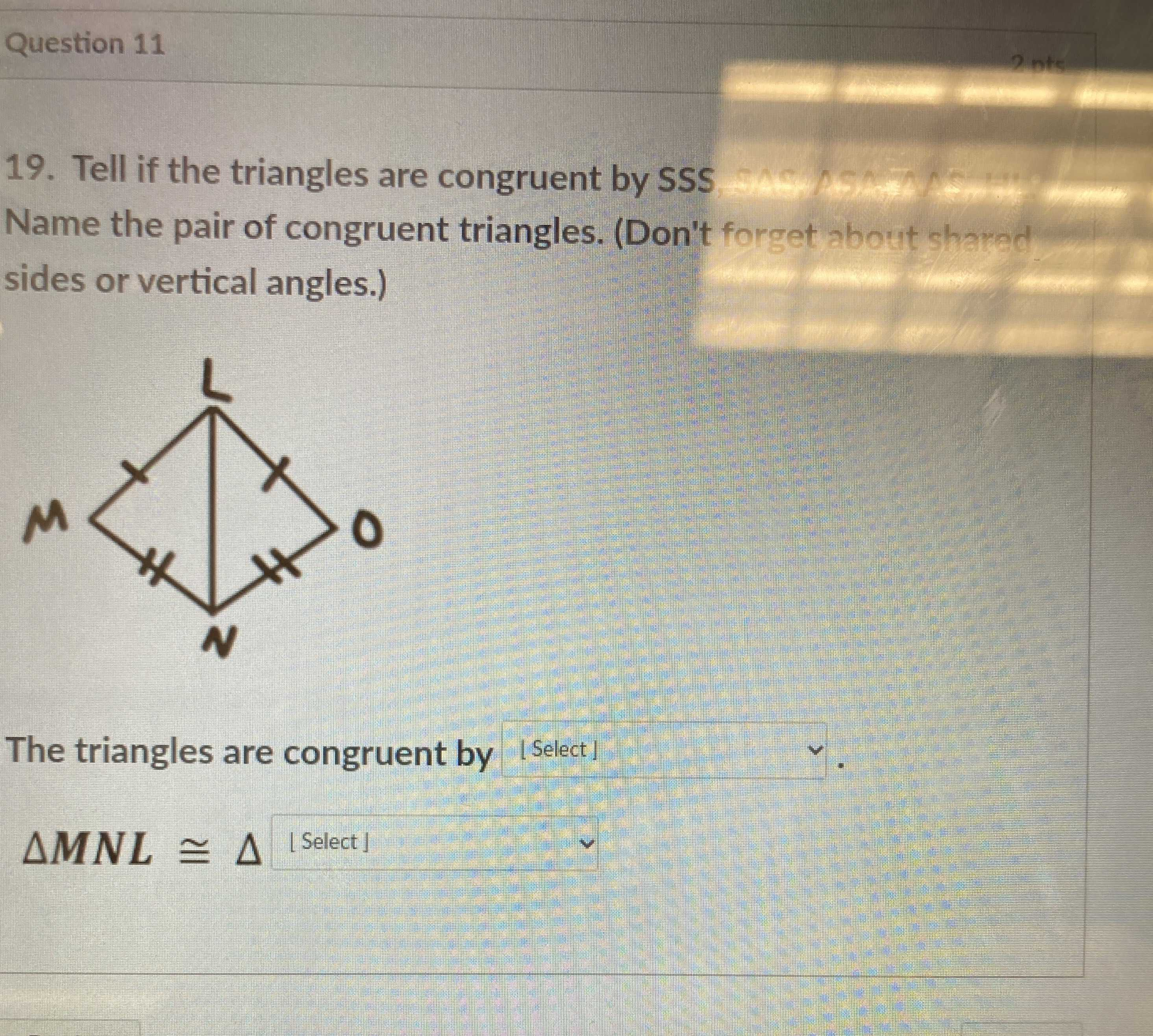### Still have math questions?19. Tell if the triangles are congruent by SSS Name the pair of congruent triangles. (Don't forget about shared sides or vertical angles.) The triangles are congruent by $$\Delta M N L \cong \Delta$$
The triangles are congruent by $$sss$$
$$\triangle M N L \cong \triangle B N L$$